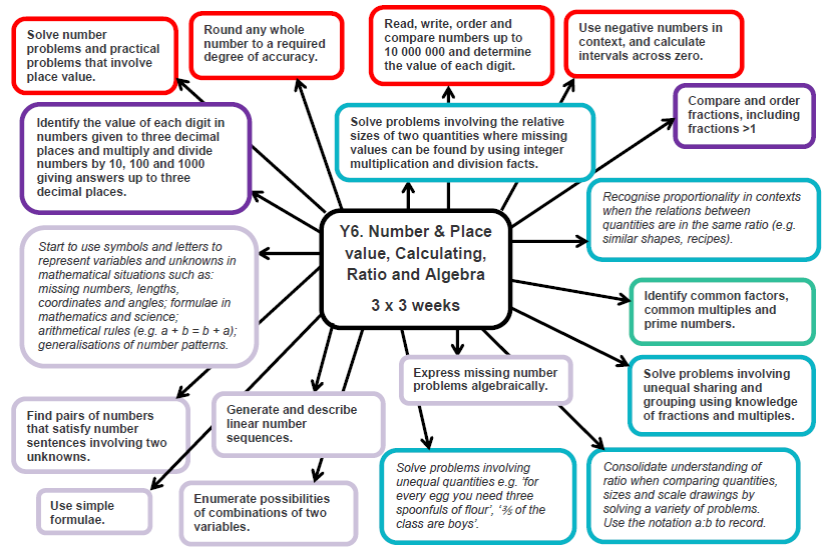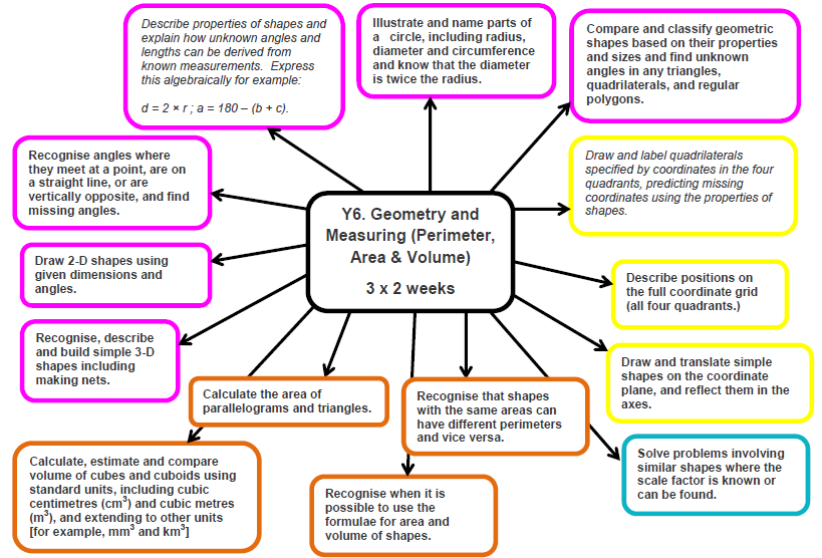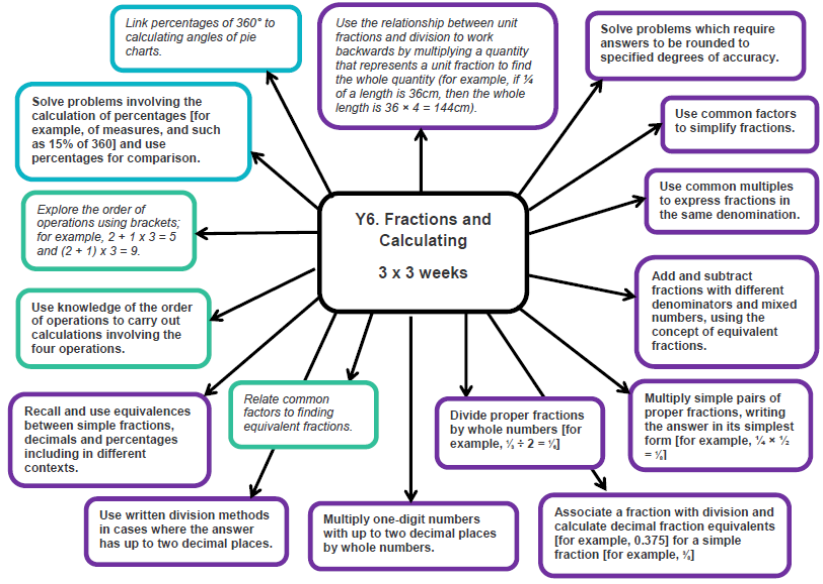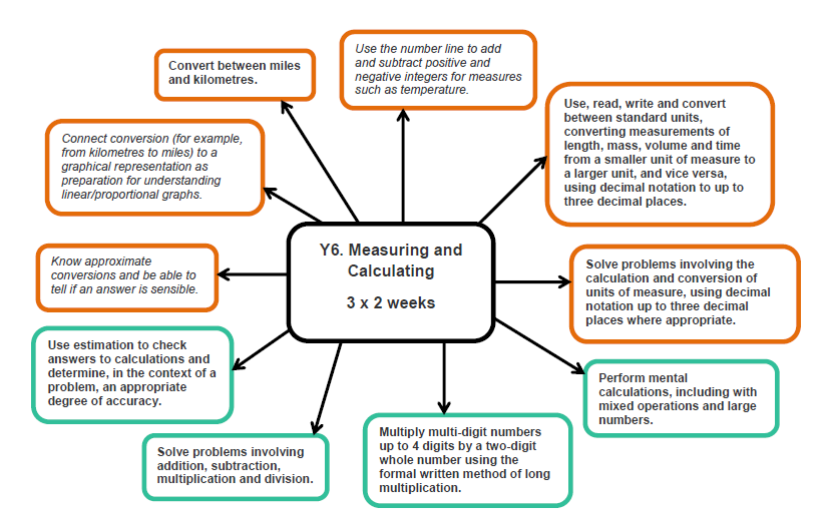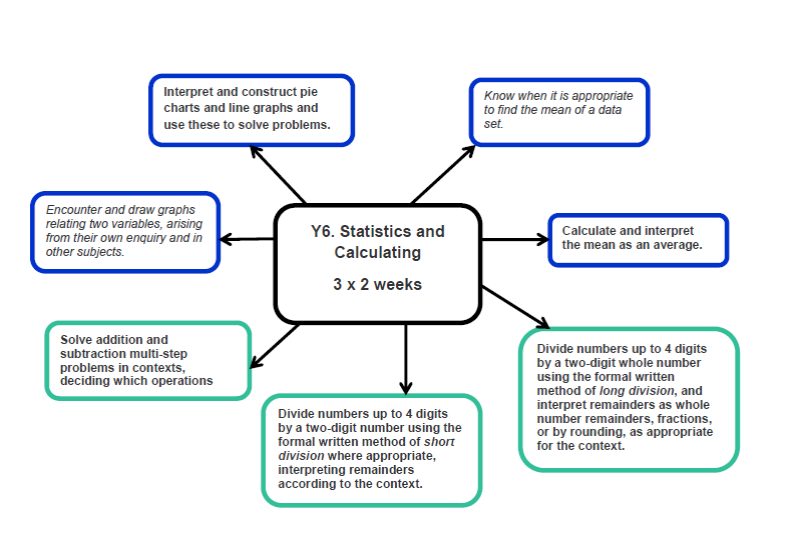#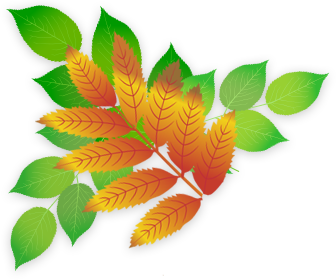# Developing Numeracy in Year 6

In Year 6 children learn to apply their mathematical skills to problem solving in a range of contexts. They work with numbers up to 10,000,000 and down to three decimal places. They learn to perform calculations using formal methods of long and short multiplication and division. They are taught how to express missing numbers in algebraic problems and use simple formulae to express number sequences and solve problems. They are shown how to generate and use formulae to calculate the area and volume of shapes. They will know properties of circles and the relationships between diameter, radius and circumference. Children are taught how to calculate percentages of quantities and relate percentages to decimals and fractions.